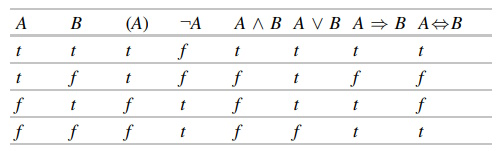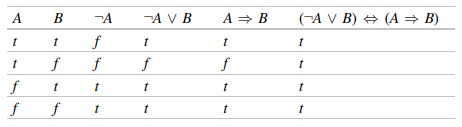#Artificial Intelligence and Propositional Logic by Wolfgang ErtelIn propositional logic, as the name suggests, propositions are connected by logical operators. The statement "the street is wet" is a proposition, as is "it is raining". These two propositions can be connected to form the new proposition

if it is raining the street is wet.

Written more formally

it is raining ⇒ the street is wet.

This notation has the advantage that the elemental propositions appear again in unaltered form. So that we can work with propositional logic precisely, we will begin with a definition of the set of all propositional logic formulas.

Syntax

Definition 2.1 Let Op = {¬,∧,∨,⇒,⇔,(,)} be the set of logic operators and ∑ a set of symbols. The sets Op, ∑ and {t,f} are pairwise disjoint. ∑ is called the signature and its elements are the proposition variables. The set of propositional logic formulas is now recursively defined:
t and f are (atomic) formulas.
All proposition variables, that is all elements from ∑, are (atomic) formulas.
If A and B are formulas, then ¬A, (A), A ∧ B, A ∨ B, A ⇒ B, A ⇔ B are also formulas.

This elegant recursive definition of the set of all formulas allows us to generate infinitely many formulas. For example, given ∑ = {A, B, C},

A ∧ B, A ∧ B ∧ C, A ∧ A ∧ A, C ∧ B ∨ A, (¬A ∧ B) ⇒ (¬C ∨ A)

are formulas. (((A)) ∨ B) is also a syntactically correct formula.

Definition 2.2 We read the symbols and operators in the following way:

 t: "true" f: "false" ¬A: "not A" (negation) A ∧ B; "A and B" (conjunction) A ∨ B: "A or B" (disjunction) A ⇒ B: "if A then B" (implication) A ⇔ B: "A if and only if B" (equivalence)

The formulas defined in this way are so far purely syntactic constructions without meaning. We are still missing the semantics.

Semantics

In propositional logic there are two truth values: t for "true" and f for "false". We begin with an example and ask ourselves whether the formula A ∧ B is true. The answer is: it depends on whether the variables A and B are true. For example, if A stands for "It is raining today" and B for "It is cold today" and these are both true, then A ∧ B is true. If, however, B represents "It is hot today" (and this is false), then A ∧ B is false.

We must obviously assign truth values that reflect the state of the world to proposition variables. Therefore we define

Definition 2.3 A mapping I : ∑ → {t, f}, which assigns a truth value to every proposition variable, is called an interpretation.

Because every proposition variable can take on two truth values, every propositional logic formula with n different variables has 2n different interpretations. We define the truth values for the basic operations by showing all possible interpretations in a truth table.Table 2.1 Definition of the logical operators by truth table

The empty formula is true for all interpretations. In order to determine the truth value for complex formulas, we must also define the order of operations for logical operators. If expressions are parenthesized, the term in the parentheses is evaluated first. For unparenthesized formulas, the priorities are ordered as follows, beginning with the strongest binding: ¬, ∧, ∨, ⇒, ⇔.

To clearly differentiate between the equivalence of formulas and syntactic equivalence, we define

Definition 2.4 Two formulas F and G are called semantically equivalent if they take on the same truth value for all interpretations. We write F ≡ G.

Semantic equivalence serves above all to be able to use the meta-language, that is, natural language, to talk about the object language, namely logic. The statement "A ≡ B" conveys that the two formulas A and B are semantically equivalent. The statement "A ⇔ B" on the other hand is a syntactic object of the formal language of propositional logic.

According to the number of interpretations in which a formula is true, we can divide formulas into the following classes.

Definition 2.5 A formula is called
Satisfiable if it is true for at least one interpretation.
Logically valid or simply valid if it is true for all interpretations. True formulas are also called tautologies.
Unsatisfiable if it is not true for any interpretation. Every interpretation that satisfies a formula is called a model of the formula.

Clearly the negation of every generally valid formula is unsatisfiable. The negation of a satisfiable, but not generally valid formula F is satisfiable.

We are now able to create truth tables for complex formulas to ascertain their truth values. We put this into action immediately using equivalences of formulas which are important in practice.

Theorem 2.1 The operations ∧, ∨ are commutative and associative, and the following equivalences are generally valid:

 ¬A∨B ⇔ A⇒B (implication) A⇒B ⇔ ¬B⇒¬A (contraposition) (A⇒B)∧ (B⇒A) ⇔ (A⇔B) (equivalence) ¬(A∧B) ⇔ ¬A∨¬B (De Morgan's law) ¬(A∨B) ⇔ ¬A∧¬B A∨(B∧C) ⇔ (A∨B)∧(A∨C) (distributive law) A∧(B∨C) ⇔ (A∧B)∨(A∧C) A∨¬A ⇔ ω (tautology) A∧¬A ⇔ f (contradiction) A∨f ⇔ A A∨ω ⇔ ω A∧f ⇔ f A∧ω ⇔ A

Proof To show the first equivalence, we calculate the truth table for ¬A ∨ B and A ⇔ B and see that the truth values for both formulas are the same for all interpretations. The formulas are therefore equivalent, and thus all the values of the last column are "t"s.Dr. Wolfgang Ertel is a professor at the Institute for Artificial Intelligence at the Ravensburg-Weingarten University of Applied Sciences, Germany.This accessible and engaging textbook presents a concise introduction to the exciting field of artificial intelligence (AI). The broad-ranging discussion covers the key subdisciplines within the field, describing practical algorithms and concrete applications in the areas of agents, logic, search, reasoning under uncertainty, machine learning, neural networks, and reinforcement learning. Fully revised and updated, this much-anticipated second edition also includes new material on deep learning.

Topics and features:

Presents an application-focused and hands-on approach to learning, with supplementary teaching resources provided at an associated website
Contains numerous study exercises and solutions, highlighted examples, definitions, theorems, and illustrative cartoons
Includes chapters on predicate logic, PROLOG, heuristic search, probabilistic reasoning, machine learning and data mining, neural networks and reinforcement learning
Reports on developments in deep learning, including applications of neural networks to generate creative content such as text, music and art
Examines performance evaluation of clustering algorithms, and presents two practical examples explaining Bayes theorem and its relevance in everyday life
Discusses search algorithms, analyzing the cycle check, explaining route planning for car navigation systems, and introducing Monte Carlo Tree Search
Includes a section in the introduction on AI and society, discussing the implications of AI on topics such as employment and transportation

Ideal for foundation courses or modules on AI, this easy-to-read textbook offers an excellent overview of the field for students of computer science and other technical disciplines, requiring no more than a high-school level of knowledge of mathematics to understand the material.

A reader says,"The book has a substantial introduction to logic that was very helpful. Overall the selection of topics was perfect for a first course in AI."

A reader says,"An excellent book for beginners. Would like to have seen code examples in python. I realize python is not much historic for AI but it is lingua franca for data science and deep learning today. Good treatment of many topics on search etc. Definitely a great resource for those just getting started in AI."